# Problem: Identify the product of radioactive decay and classify the given nuclear reaction accordingly (α decay, β decay or γ decay).

###### FREE Expert Solution
100% (440 ratings)
###### FREE Expert Solution
100% (440 ratings)###### Problem Details

Identify the product of radioactive decay and classify the given nuclear reaction accordingly (α decay, β decay or γ decay).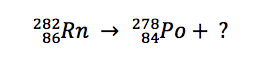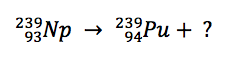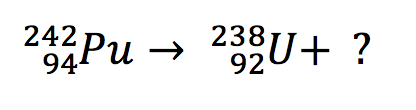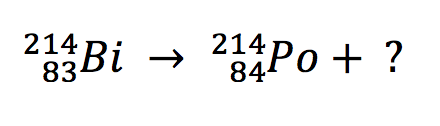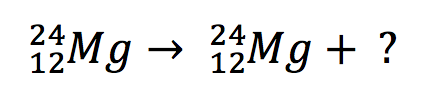What scientific concept do you need to know in order to solve this problem?

Our tutors have indicated that to solve this problem you will need to apply the Radioactive Decay concept. You can view video lessons to learn Radioactive Decay . Or if you need more Radioactive Decay practice, you can also practice Radioactive Decay practice problems.

What is the difficulty of this problem?

Our tutors rated the difficulty ofIdentify the product of radioactive decay and classify the g...as medium difficulty.

How long does this problem take to solve?

Our expert Chemistry tutor, Dasha took 4 minutes and 36 seconds to solve this problem. You can follow their steps in the video explanation above.

What professor is this problem relevant for?

Based on our data, we think this problem is relevant for Professor Brummett's class at IOWA.